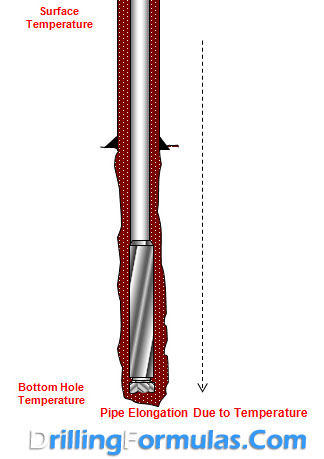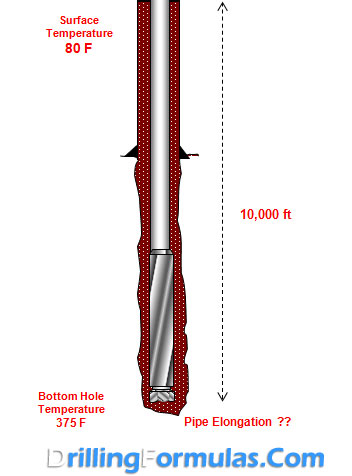# Drill Pipe Elongation Due to Temperature

Drill pipe elongation will occur because the higher bottom hole temperature.Rule of Thumb

Pipe will elongate approximately 0.83 inc per 100 ft of length, per 100 degree F increase in Temperature.

We need to know the surface temperature and bottom hole temperature in order to determine pipe elongation.

The following formulas are used to determine pipe elongation.

Average Temperature = (Bottom Hole Temperature + Surface Temperature) ÷ 2

∆ Temperature = Average Temperature – Surface Temperature

Pipe Elongation = (L ÷ 100) × (∆ Temperature ÷ 100) × 0.83

Where

Average Temperature, Bottom Hole Temperature and Surface Temperature are in F.

Pipe Elongation is in inch.

L is total length in feet.

Example

Determine pipe elongation from the following information.

Total length of pipe is 10,000 ft.

Surface temperature is 80 F.

Bottom hole temperature is 375 F.Average Temperature = (80 + 375) ÷ 2 = 227.5 F

∆ Temperature = 227.5 – 80 = 147.5 F

Pipe Elongation = (10,000 ÷ 100) × (147.5 ÷ 100) × 0.83 = 122.43 inch

Share the joyWorking in the oil field and loving to share knowledge.

### 5 Responses to Drill Pipe Elongation Due to Temperature

1.Arsa says:

Thanks a lot for your useful notes.
please work other useful rule of thumbs in drilling operation.

2.Cristi says:

Great formula guys!
Does it applies to metric units, also?

All the best,
Cristi

•DrillingFormulas.Com says:

No. We don’t have it in the metric unit at the moment.

3.abdel hakiem el nahhal says:

it is very usefull equations and practical , thanks a lot

4.Manuel says:

Thank you very much*

This site uses Akismet to reduce spam. Learn how your comment data is processed.